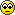SB Physics Help Room Sucks!
Would you like to react to this message? Create an account in a few clicks or log in to continue.

# maple ta quiz 24.1##maple ta quiz 24.1

1. mass = density * volume (cm^3)
mass/55.8u = # of moles
N (iron atoms) = 6.02E23 * #of moles

2. theta = (arc sin (wavelength/(2* distance)))*2

3. magnetic field = (electric field/velocity)

4. radius= ((velocity * mass)/(1.6E-19 * magnetic field))

Enjoy ya spring break!!

hmmmm..
Guest

##Q#2

i am having problems with question #2 the answer keeps coming up incorrect. Are you sure that is how you slove it?

phy122
Guest

##Question #1

for number one, the density is given and i dont know which one is the volume cuz its not given??? i dont quite understand the fourmula for number one and my atom is uranium???

guest 00
Guest

##Re: maple ta quiz 24.1

phy122 wrote:i am having problems with question #2 the answer keeps coming up incorrect. Are you sure that is how you slove it?

The solution is correct.

angle = (arc sin (wavelength/(2* distance)))*2

Remember, arc sin means sin^-1.

Also, the entire right side of the equation is multiplied by 2 (i.e. after you get the arc sin of (lambda/2*d)).

DJ

Posts : 28
Join date : 2009-03-17

##Re: maple ta quiz 24.1

guest 00 wrote:for number one, the density is given and i dont know which one is the volume cuz its not given??? i dont quite understand the fourmula for number one and my atom is uranium???

Everyone has Uranium.

Remember - volume is height * length * width for a cube. Thus, the volume is simply the number in cm to the third power.

The formulas:

First, find mass using this equation: mass = density * volume

Then, divide the mass by 55.8 molar mass to find the number of moles in the cube: mass/55.8u = # of moles

Finally, find the number of atoms by multiplying by Avogadro's number: # atoms= 6.02E23 * #of moles.

Very simple.

DJ

Posts : 28
Join date : 2009-03-17

##Question 2

Hey guys i Keep getting this wrong. My question is
"2) X-Rays with a wavelength of 0.1654 nm are scattered from a crystal with a distance of 0.2443 nm between the crystal planes. At which scattering angle does the first order diffraction maximum occur ? (sheet 9,11, the scattering angle is not the same angle which occurs in the maxumum condition!)"
Ok well I did eevrything that was shown and I get a value of 0.69068

MapleTA says Im wrong... Anyone have a clue what im doing wrong? Help would be much appreciatedMoney23
Guest

##Nevermind

Never mind. I just forgot to switch to degree mode.Money23
Guest

##Re: maple ta quiz 24.1